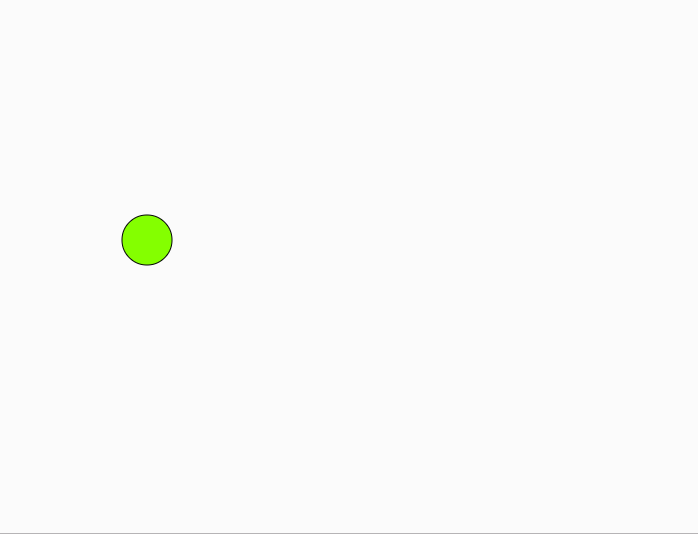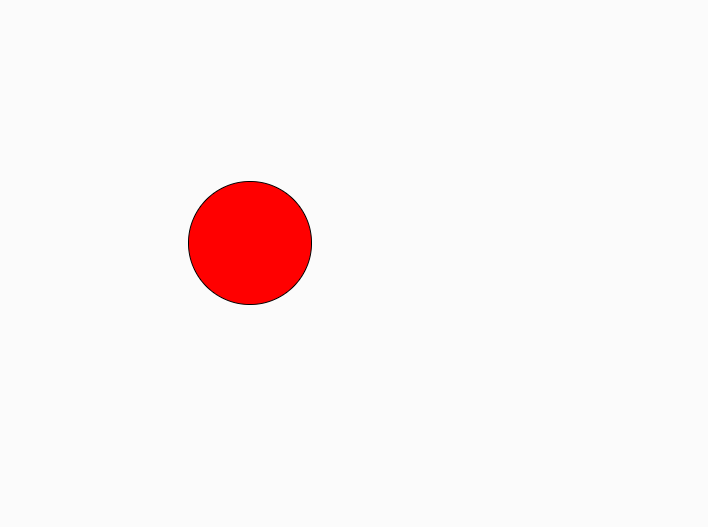# p5.js | Keyboard | keyIsDown()

• Last Updated : 16 Apr, 2019

The keyIsDown() function in p5.js checks the key’s current status that key is down, i.e. pressed. It can be used if you have a movable object, and you want several keys to be able to affect its behavior simultaneously, such as moving a sprite diagonally.

Syntax:

```keyIsDown()
```

Below program illustrates the keyIsDown() function in p5.js:
Example-1:

 `let x = 100;``let y = 100;`` ` `function` `setup() {``   ` `    ``// create canvas of size 600*600``    ``createCanvas(600, 600);``}`` ` `function` `draw() {``   ` `    ``// fill color``    ``fill(x, y, x - y);`` ` `    ``if` `(keyIsDown(LEFT_ARROW)) {``        ``x -= 5;``    ``}`` ` `    ``if` `(keyIsDown(RIGHT_ARROW)) {``        ``x += 5;``    ``}`` ` `    ``if` `(keyIsDown(UP_ARROW)) {``        ``y -= 5;``    ``}`` ` `    ``if` `(keyIsDown(DOWN_ARROW)) {``        ``y += 5;``    ``}`` ` `    ``clear();``    ``ellipse(x, y, 50, 50);``}`

Output:Example-2:

 `let diameter = 30;`` ` `function` `setup() {``   ` `    ``// Create canvas of size 600*600``    ``createCanvas(600, 600);``}`` ` `function` `draw() {``   ` `    ``// 107 and 187 are keyCodes for "+"``    ``if` `(keyIsDown(107) || keyIsDown(187)) {``        ``diameter += 1;``    ``}`` ` `    ``// 109 and 189 are keyCodes for "-"``    ``if` `(keyIsDown(109) || keyIsDown(189)) {``        ``diameter -= 1;``    ``}`` ` `    ``clear();``    ``fill(255, 0, 0);``    ``ellipse(width / 2, height / 2, diameter, diameter);``}`

Output:Reference: https://p5js.org/reference/#/p5/keyIsDown

My Personal Notes arrow_drop_up